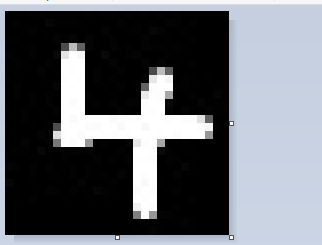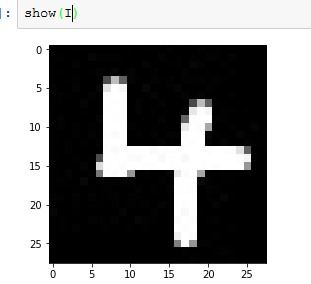# Beginners tutorial: Predict your number with MNIST

Should this be moved to another forum?
I’m mainly writing this to see if you agrees with my approach. I focus on getting a custom image loaded, transformed and predicted. This is the part a lot of beginners (myself included) struggle with. I’m using the NN from the MNIST notebook. (~/courses/ml1/lesson4-mnist_sgd.ipynb)

Step 1 Draw a pretty number.Step 2 Load it.

``````import PIL
I = np.asarray(PIL.Image.open(path+'4.bmp')) #Depending on the image format you will have to remove two color channels
I.shape
>>>(28, 28)
``````

You can verify it with show(I):Step 3 Transform and normalize and normalize some more:

``````four = I.flatten()
type(four),four.shape
>>>(numpy.ndarray, (784,))

#normalize, scale 0-255 to 0.0-1.0
four = (four-min(four))/(max(four)-min(four))
``````

I use the mean and std from the MNIST dataset cor consistency. My ‘number 4’ array is not as close to mean=0, std=1 and I would like, but I don’t know if that is a problem.

``````four = (four-mean)/std
four.mean(), four.std()
>>>(0.13384368359694745, 1.1896541432197534)
``````

Step 4 Convert the numpy array to a tensor, then a ‘Variable’.

``````Vfour = V(T(four))
type(Vfour), Vfour.shape #single column

Vfour = Vfour.resize(1,784)
Vfour.shape
>>>torch.Size([1, 784])
``````

Why transpose/permute/resize? Because the model expects 784 columns, not rows.

Step 5 Predict!

``````predictFour = net2(Vfour).exp()
predictFour
>>>Variable containing:
Columns 0 to 5
7.8360e-16  1.2982e-25  4.6790e-20  5.4591e-16  1.0000e+00  2.2921e-19

Columns 6 to 9
2.3876e-13  3.3228e-15  1.3799e-14  4.0984e-10
[torch.cuda.FloatTensor of size 1x10 (GPU 0)]

predictFour.max(1)
>>>Variable containing:
4
[torch.cuda.LongTensor of size 1 (GPU 0)]
``````

Thats what I did. I would love feedback on my approach.

1 Like